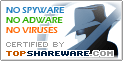# Ordinary Differential Equations 1.00

SNAPSHOT

Solves ordinary differential equations

Windows AllPlatform :
USD \$15Price :
2.33 MBFile Size :
ScreenshotScreenshot :Popularity :Solves boundary-value or initial-value problems involving nonlinear or linear ordinary differential equations of any order, or systems of such. The conditions may also be linear or nonlinear. The solution will be a finite power or trigonometric series. You have one-click control of the graph with zooming, panning, centering, etc. The solution may be studied by the POWER & TRIG SERIES program to find roots, extrema, derivatives, integral, graph.

• Functions
Visualizes and studies functions of one variable to find roots, extrema, integral, derivatives, graph. Results can be saved or printed. You have control of the graph with zooming, panning, centering.
• Linear Algebra
Computations associated with matrices
• Equation graph plotter - EqPlot
Eqplot plots 2D graphs of complex equations.
• BaKoMa TeX
BaKoMa TeX with TeX Word - True WYSIWYG LaTeX Editor.
Multipurpose analytical calculator
• XCalc
Easy to use sizable, skinned calculator.
• jExacter
jExacter (Java) - a high precision calculator
• Optimumcut-1D
Optimumcut-1D Stand-Alone Cutting Optimizer
• Jobber PC
Ultimate Construction Calculator for PocketPC
• VIGraph
Graph builder for your txt data files
• Function Grapher
Graph maker to create 2d, 2.5d and 3D function graphs and animations.
• My Calculator
Fast, easy to use and well equipped mathematical expression evaluator